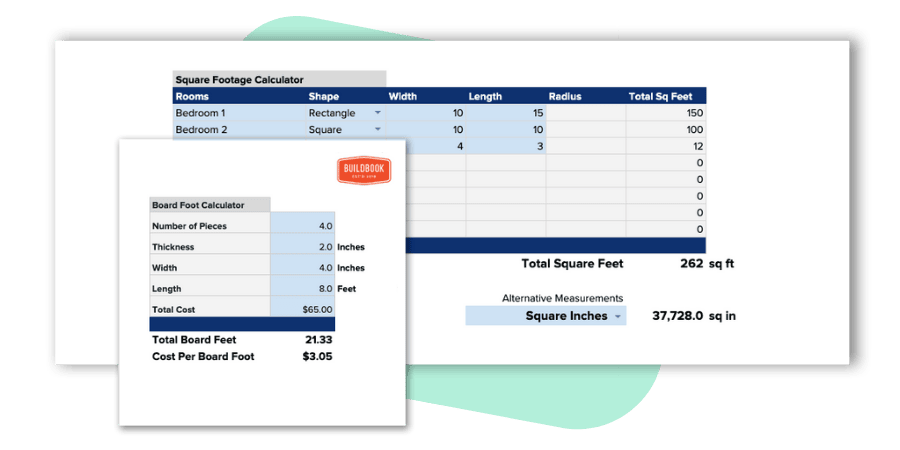# Concrete CalculatorCalculate the amount of concrete needed in cubic feet, yards, and bags for a concrete slab

## Looking for an even better free concrete calculator for your construction business?

This collection of construction calculators is 100% free to download, copy, and use as your own. You're free to modify and/or adjust any calculator to best fit the needs of you or your business.This concrete calculator allows you to estimate how much concrete you need based on the cubic feet and cubic yards for a defined area. Additionally, using the three most common sizes of cement bags — 40, 50 and 60 pound — this calculator will help you determine the number of bags you need for your project.

## How to calculate the volume of concrete you need

Concrete is most often sold based on cubic yards, so in order to find out how much concrete you need, it's easiest to begin by calculating the cubic feet of your area.

Cubic feet formula: Length * width * depth/thickness

For example, if your slab is 10' wide and 10' long with 1' of depth, the volume of your slab would be 100 cubic feet (10x10x1 =100). Next we'll convert our cubic feet into cubic yards.

Cubic yard formula: total cubic feet * .037

It's important to note that a cubic yard is different than a standard yard. While there are 3 feet to standard yard, there are 27 feet to a cubic yard (3 x 3 x 3). To find your total cubic yards, you can either divide 100 by 27 or multiply 100 by .037 as shown in the formula above (.037 = 1 / 27)

In this example, you end up with 3.70 cubic yards for your slab (100 x .037).

## How to estimate the number of cement bags you need

To estimate how many bags of cement you will need for a project, you'll need to know two things:

1. The size of the cement bag
2. The cubic yards or your slab

With those two data points, you can easily estimate the number of bags you'll need based on customary measurement standards of bags per cubic yard.

Cement bags needed: total cubic yards * cubic yards per bag

The standard measurements per bag for the three most common sizes are as follows:

• 40 lb bag: .011
• 60 lb bag: .017
• 80 lb bag: .022

Using the previous example of a 10 x 10 slab with a volume of 3.70 cubic yards, the number of bags you would need based on each bag size are as follows:

• 40 lb bag: 3.70 * .011 = 366 bags
• 60 lb bag: 3.70 * .017 = 218 bags
• 80 lb bag: 3.70 * .022 = 168 bags

Keep in mind that your result is an estimate based on standard measurements and doesn't account for any potential waste or spillage.